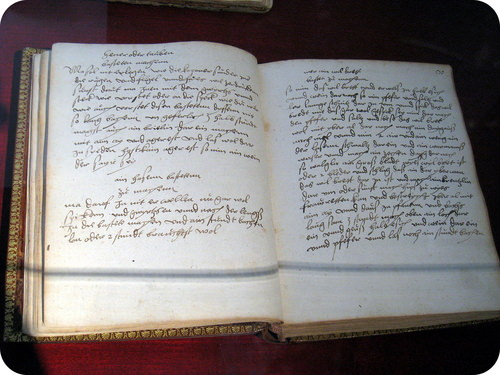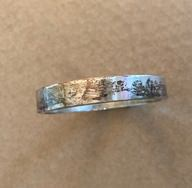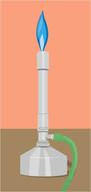# 5.1: Word Equations

$$\newcommand{\vecs}{\overset { \rightharpoonup} {\mathbf{#1}} }$$ $$\newcommand{\vecd}{\overset{-\!-\!\rightharpoonup}{\vphantom{a}\smash {#1}}}$$$$\newcommand{\id}{\mathrm{id}}$$ $$\newcommand{\Span}{\mathrm{span}}$$ $$\newcommand{\kernel}{\mathrm{null}\,}$$ $$\newcommand{\range}{\mathrm{range}\,}$$ $$\newcommand{\RealPart}{\mathrm{Re}}$$ $$\newcommand{\ImaginaryPart}{\mathrm{Im}}$$ $$\newcommand{\Argument}{\mathrm{Arg}}$$ $$\newcommand{\norm}{\| #1 \|}$$ $$\newcommand{\inner}{\langle #1, #2 \rangle}$$ $$\newcommand{\Span}{\mathrm{span}}$$ $$\newcommand{\id}{\mathrm{id}}$$ $$\newcommand{\Span}{\mathrm{span}}$$ $$\newcommand{\kernel}{\mathrm{null}\,}$$ $$\newcommand{\range}{\mathrm{range}\,}$$ $$\newcommand{\RealPart}{\mathrm{Re}}$$ $$\newcommand{\ImaginaryPart}{\mathrm{Im}}$$ $$\newcommand{\Argument}{\mathrm{Arg}}$$ $$\newcommand{\norm}{\| #1 \|}$$ $$\newcommand{\inner}{\langle #1, #2 \rangle}$$ $$\newcommand{\Span}{\mathrm{span}}$$$$\newcommand{\AA}{\unicode[.8,0]{x212B}}$$Figure $$\PageIndex{1}$$ (Credit: User:Daderot/Wikimedia Commons; Source: http://commons.wikimedia.org/wiki/File:Innsbruck_-_Schloss_Ambras_-_cookbook_of_Philippine_Welser.jpg(opens in new window); License: Public Domain)

### What's for Dinner?

Various ways of recording recipes have developed over the centuries. The cookbook shown above was written by a woman who probably collected all her own recipes. Later, printed cookbooks became available (even guys had no excuse for not being able to cook). Today we can find recipes on a number of internet sites and can quickly search for information on how to cook anything we want. Reading a recipe sometimes requires we understand a few codes and symbols (what’s the difference between a tsp and a Tsp?), but the information on what we start with and what we end up with is there.

## Writing Chemical Equations

Chemical reactions are occurring all around us. Plants use sunlight to drive their photosynthetic process and produce energy. Cars and other vehicles burn gasoline in order to power their engines. Batteries use electrochemical reactions to produce energy and power many everyday devices. Many chemical reactions are going on within the human body as well, particularly during the digestion of food.

In math class, you have written and solved many mathematical equations. Chemists keep track of chemical reactions by writing equations as well. In any chemical reaction, one or more substances—called reactants—are converted into one or more new substances—called products. The general form of the equation for such a process looks like this:

$\text{Reactants} \rightarrow \text{Products}\nonumber$

Unlike a math equation, a chemical equation does not use an equal sign. Instead, the arrow is called a yield sign and so the equation is described as "reactants yield products".

## Word Equations

You can describe a chemical reaction by writing a word equation. When silver metal is exposed to sulfur, it reacts to form silver sulfide. Silver sulfide is commonly known as tarnish and turns the surface of silver objects dark and streaky black (see figure below). The sulfur that contributes to tarnish can come from traces of sulfur in the air, or from foods such as eggs. The word equation for the process is:

$\text{Silver} + \text{sulfur} \rightarrow \text{Silver sulfide}\nonumber$

The silver and the sulfur are the reactants in the equation, while the silver sulfide is the product.Figure $$\PageIndex{2}$$: You can see dark spots of tarnished (silver sulfide) forming on this ring as it reacts with sulfur compounds in the air. (Credit: CK-12 Foundation; Source: CK-12 Foundation; License: CK-12 Curriculum Materials license)

Another common chemical reaction is the burning of methane gas. Methane is the major component of natural gas and is commonly burned on a gas stove or in a Bunsen burner (figure below). Burning is a chemical reaction in which some type of fuel is reacted with oxygen gas. The products of the reaction in the burning of methane, as well as other fuels, are carbon dioxide and water. The word equation for this reaction is:

$\text{Methane} + \text{oxygen} \rightarrow \text{carbon dioxide} + \text{water}\nonumber$Figure $$\PageIndex{3}$$: A Bunsen burner is commonly used to heat substances in a chemistry lab. Methane is reacted with oxygen to form carbon dioxide and water. (Credit: CK-12 Foundation; Source: CK-12 Foundation; License: CK-12 Curriculum Materials license)

Word equations can be very useful, but do have one major drawback—they cannot be used for any quantitative work. A word equation does not tell how many moles of each material are needed, or how many moles of product are formed.

## Summary

• Word equations are used to describe the conversion of reactants to products.

## Review

1. Write the generic form of a chemical reaction.
2. What are reactants?
3. What are products?

5.1: Word Equations is shared under a CC BY-NC license and was authored, remixed, and/or curated by LibreTexts.